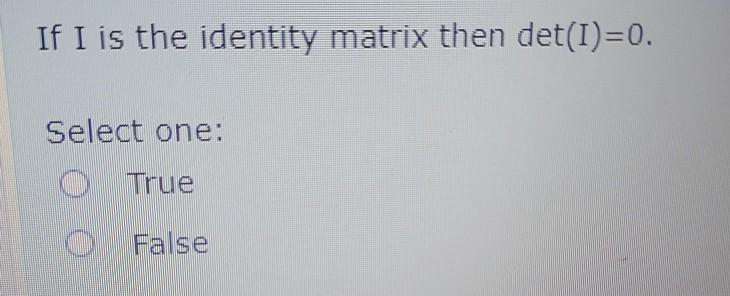Create an Account

Home / Questions / If I is the identity matrix then det(I)=0. Select one: O True 0 False

If I is the identity matrix then det(I)=0. Select one: O True 0 False

If I is the identity matrix then det(I)=0. Select one: O True 0 FalseMay 30 2021 View more View LessSubscribe To Get Solution